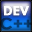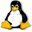Home Latest News Archive Tutorials C++ 2 3 4 Win32 Java OpenGL GLUT OpenGL ES 2 3 4 DirectX VB.NET HTML Photoshop 3dsmax Forums Projects Modelling Links About Us Search Recent Tutorials C / C++ Win32 OpenGL OpenGL ES GLUT## Multi-Dimensional Arrays

Tutorials > C++ > Multi-Dimensional Arrays

### What are Multi-Dimensional Arrays?

You were given an introduction to arrays in tutorial 7. This tutorial dealt only with 1-dimensional arrays. An example of a 1-dimensional array is given below.

 1 2 3 4

A 2-dimensional array can be seen as an array of arrays ie. a table.

 1 2 3 4 5 6 7 8 9 10 11 12 13 14 15 16

Imagine each row as being a separate array. This concept will become more familiar further on. A 3-dimensional array can be seen as a number of 2-dimensional arrays layered on top of each other. This is used in certain application such as 3D graphics where 3D coordinates need to be specified. You can specify any N-dimensional array in C++. Generally you would never exceed 4 dimensions.

### How are Multi-Dimensional Arrays declared?

Remember that square brackets are placed after the variable name to specify an array. Additional sets of square brackets can be placed to specify additional dimensions.

Contents of main.cpp :

```#include <iostream>
#include <stdlib.h>

using namespace std;

int main()
{
```

Below, we define a 2-dimensional matrix. You can specify the size of each dimension within brackets. If you are initializing the matrix at the same time of declaration, you can leave out the size in the first bracket and it will automatically be calculated. You can use curly brackets to see the rows more easily.

```	int matrix[] = {
{1, 2, 3, 4},
{5, 6, 7, 8},
{9, 10, 11, 12}
};
```

The number of elements in the multi-dimensional array can be calculated in the normal manner.

```	int numElements =
sizeof(matrix) / sizeof(int);

cout << "Elements : "
<< numElements << endl;
```

Remember I said that you can think of a 2-dimensional array as an array of arrays? If you output the value of matrix, you will find that it returns a memory address and not a normal number. This is because matrix points to the first element of the first row, ie. matrix.

```	cout << matrix << endl;
```

There will be a great number of times where you will need to output or traverse the values of an N-dimensional array. This can be achieved using N for loops. The code below outputs the values in the 2-dimensional array. The first for loop loops through each row and the second for loop looks at each value in the row. An endline is placed at the end of each row.

```	for (int row = 0; row < 3; row++)
{
for (int col = 0; col < 4; col++)
cout << matrix[row][col] << " ";

cout << endl;
}
```

A quick example of declaring a 3-dimensional array is given below.

```	bool room;
```

Elements of the array can be accessed as per normal. If the middle bracket specifies the height of the room, then the value in the center of the room on the floor is set to true. This could be used to specify what parts of the room are occupied.

```	room = true;

system("pause");

return 0;
}```

You should now have an understanding of multi-dimensional arrays and how they can be used effectively.

Please let me know of any comments you may have : Contact Me

 Source Files :< Tutorial 24 - Macros Tutorial 26 - Typedef >